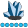plf515
Lapis Lazuli | Level 10

## Adding Summary Statistics to VBOX SGPANEL

Hello

Using SAS 9.4 TS 1M4 on Windows

I have a data set that contains variables _NODE_ and mpg, e.g

Obs MPG _Node_ _Leaf_ P_MPG1234
 18 9 2 14.0119 15 9 2 14.0119 18 9 2 14.0119 16 9 2 14.0119

I would like to make a box plot by node but add a box for all the nodes.

I tried

``````proc sgplot data = tree.mpgout;
vbox mpg /group = _node_;
vbox mpg/boxwidth= 0.1;
run;``````

but this put the second box in the middle, blotting out other boxes.  I didn't see a way to set the location of the boxes.

How can I make this plot? THanks

1 ACCEPTED SOLUTION

Accepted Solutions

## Re: Adding Summary Statistics to VBOX SGPANEL

To get all of this into a single graph probably the easiest is to modify your data so that you have your existing data plus a duplicate that has a _node_ value to indicate the "whole data".

That code would look something like:

```data tree.vboxplot;
set tree.mpgout
tree.mpgout(in=in2)
;
if in2 then _node_='Total';
run;```

You have no indication whether _node_ is numeric or character so I can't provide anything guaranteed to work but that should give you an idea. If you want the whole data on the left set a node value smaller or earlier in the sort order, on the right set a value larger or after the others.

The plot code would then use the new set and drop the second vbox statement:

```proc sgplot data = tree.vboxplot;
vbox mpg /group = _node_;
run;```

## Re: Adding Summary Statistics to VBOX SGPANEL

To get all of this into a single graph probably the easiest is to modify your data so that you have your existing data plus a duplicate that has a _node_ value to indicate the "whole data".

That code would look something like:

```data tree.vboxplot;
set tree.mpgout
tree.mpgout(in=in2)
;
if in2 then _node_='Total';
run;```

You have no indication whether _node_ is numeric or character so I can't provide anything guaranteed to work but that should give you an idea. If you want the whole data on the left set a node value smaller or earlier in the sort order, on the right set a value larger or after the others.

The plot code would then use the new set and drop the second vbox statement:

```proc sgplot data = tree.vboxplot;
vbox mpg /group = _node_;
run;```

Discussion stats